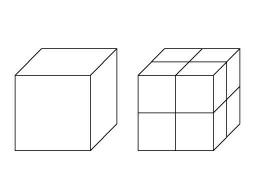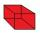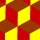# Cube zoom

How many percents do we increase the volume and surface of the cube if we magnify its edge by 38 %?

Δ V =  162.8 %
Δ S =  90.4 %

### Step-by-step explanation:Did you find an error or inaccuracy? Feel free to write us. Thank you!Tips to related online calculators
Tip: Our volume units converter will help you with the conversion of volume units.

## Related math problems and questions:

• Volume and body diagonalCalculate how much the volume and body diagonal of the cuboid decrease if we reduce each of its three edges a, b, c by 18%?
• Surface area 2Calculate how many % reduce the surface area of the cube is we reduced length of each edge by 10%.
• Reduce of the volumeCalculate how many % reduce the volume of the cube is we reduced length of each edge by 10%.
• Content area and percentsDetermine what percentage is smaller cube surface, when the surface area of the wall decreases by 25%.
• Cube edgesIf the edge length of the cube increases by 50%, how does the volume of this cube increase?
• Magnified cubeIf the lengths of the cube's edges are extended by 5 cm, its volume will increase by 485 cm3. Determine the surface of both the original and the magnified cube.
• Square sideIf we enlarge the square side a = 5m, its area will increase by 10,25%. How many percent will the side of the square increase? How many percent will it increase the circumference of the square?
• HoleIn the center of the cube with edge 14 cm we will drill cylinder shape hole. Volume of the hole must be 27% of the cube. What drill diameter should be chosen?
• Cube 6Volume of the cube is 216 cm3, calculate its surface area.
• Cube surface and volumeThe surface of the cube is 500 cm2, how much cm3 will be its volume?
• Special cubeCalculate the edge of cube, if its surface and its volume is numerically equal number.
• The cubeThe cube has an edge of 12 dm. The second cube has an edge exactly 20% longer. How many % is more water in the second cube than in the first cube, if the first cube is full to 3/4 and the second to 3/8?
• Two boxes-cubesTwo boxes cube with edges a=38 cm and b = 81 cm is to be replaced by one cube-shaped box (same overall volume). How long will be its edge?
• Area to volumeIf the surface area of a cube is 486, find its volume.
• CylindersThe area of the side of two cylinders is the same rectangle of 33 mm × 18 mm. Which cylinder has a larger volume and by how much?
• LatheFrom the cube of edge, 37 cm was a lathed maximum cylinder. What percentage of the cube is left as waste after lathed?
• Cube wallThe surface of the first cube wall is 64 m2. The second cube area is 40% of the surface of the first cube. Determine the length of the edge of the second cube (x).